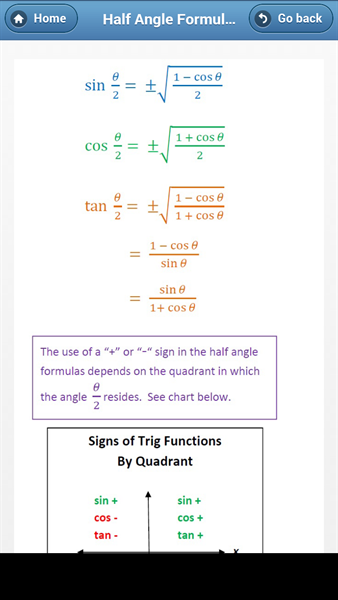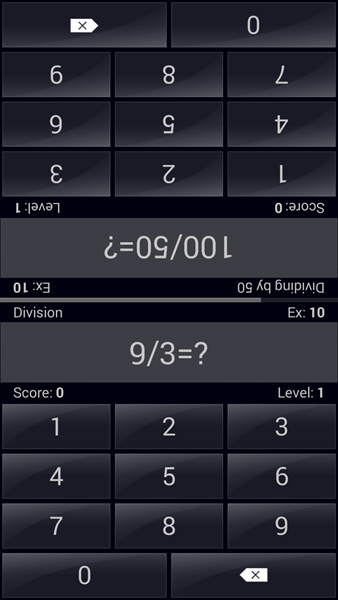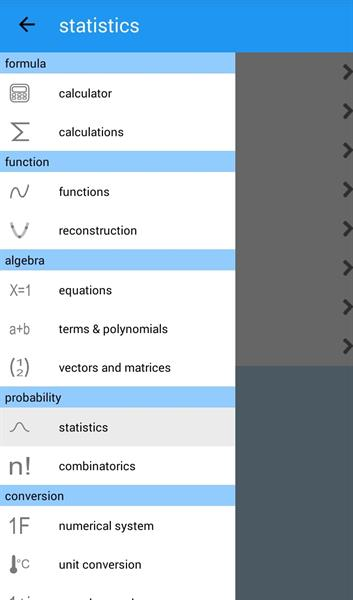Mathematics is the most boring or scary subject for most students. But let me tell you that mathematics is the most beautiful and interesting subject out there. You just need to study it in the right way. I have mentioned some Android applications in this article that can improve your mathematics like never before. Read on to know more.

## Introduction

Mathematics is an extremely important thing in our lives. Though some people hate mathematics or are afraid of mathematics, it is a subject that you have to deal with anyway in most of the areas of your lives. So, wouldn't it be better if you study at least some of the basics of mathematics? Okay, so if you are ready to learn, you have come to the right place. I will provide you with some resources which can be useful to you in learning and getting good at mathematics. There are some applications in Android which are designed for learning and revising the concepts of mathematics.
These applications are perfectly designed and developed in such a way that it is easy for you to get started with their easy to use user interface. So, without much introduction let's get right into it.

## All Math FormulaThis is a perfect application if you are already a math student and need to quickly find out a formula. All Math Formula has four broad categories which are Algebra, Geometry, Trigonometry and Calculus. Each of these categories contain sub-categories which contain almost all the formulas and equations that are used commonly by engineering students. So, if you are an engineering student this application can be of a great help to you.
Some of the formulas that are included in this application are Circle, Ellipse, Hyperbola, Pythagorean Identities, Limits, Mean Value Theorems, Newton's Method, Common Integrals, Approximating Definite Integrals and more. There are a lot of formulas that you commonly need in your studies.
This application is developed by Tech-tweets.

## Math TricksMath Tricks is an application which can help you increase your speed in solving mathematical questions. Some tips and tricks are shown in this application for all the basic operations to do the calculations as fast as possible. You can learn these tricks and impress your friends and show them how fast you can do the calculations.
In this application you have different modes which are Single Game where you can start solving challenges by going to different levels, Multiplayer where you can play real time with other users using the internet, Joint Game where your screen is split into two mirror sections where you can play with your opponent sitting right in front of you on your own phone, Training mode where you are taught some techniques and tricks to do calculations, By heart where you can learn multiplication tables and Settings to change the preferences of the application. This application is developed by Antoni.

## MathematicsThis application is kind of like an advanced calculator. You can calculate pretty much anything using this application. Some of the amazing things that you can do with this calculator are formula calculations with variables, sums, products and sequences, solve equations (linear, quadratic, cubic), factorization, differential and integral calculus, unit conversion, complex number calculations, statistics and many more.
One of the features that I like in this application is that you can plot graph using an equation. This will help you visualize the equation and let you know how things are working under the hood. You also have the ability to plot in 3D with this amazing application.
You also have the option of calculating vectors, determinants and matrices which are not available in most of the common calculators. These features will definitely make your mathematical life a lot easier and fun as well.
Multiple languages are supported for this application like German, Italian, Portuguese, etc. This application is developed by daboApps.

## Complete MathematicsComplete Mathematics, as the name tells is an application which has pretty much anything that you would think of about mathematics. This application is kind of like the application that contains most of the topics in the applications discussed above. It also has tutorials and notes which you can refer to and learn new concepts. There are also quizzes and other such things which will help you hone your skills and make you a better mathematician. It covers all the basic and high school mathematical topics in a simple and elegant way. Now you can't complain or make excuses about learning mathematics if you are a student. Trust me, you will love learning mathematics with applications as these.
This application has the following categories:
Tutorials: This section of the application contains a ton of tutorials around basic concepts of high school and basic mathematics. You can browse through them and learn them. Just select one of the topics and you will be guided to its tutorial.

Formulas: Some of the formulas available in this section are regarding Constants, Quadratic Equation, Indices, Logarithms, Trigonometry, Circle and many more.
Calculation: This section deals with different types of calculations. You can enter your inputs and select the correct category and get the output in a flash. Some of the categories in this section are Simultaneous Equations, Quadratic Equations, Trigonometry, Fibonacci Sequence and more.

Theory Questions: In this section you are provided with different theoretical questions on mathematics and you have to answer them. These are the questions that are asked in some entrance examinations. There is also a button called Find Answers which you can touch if you are having trouble finding the answer to the question.

Quiz: There are three categories in this section. Each of these categories contains 20 questions each which have four options and you have to select the correct one from them.

Dictionary: This section of the application contains all the common terms that are used in mathematics. You can select or search for any term and touch on it to know what it is and the meaning of the term.

Scientific Calculator: Scientific calculator can do much of the college level calculations including trigonometric functions.

Graphing Calculator: With Graphing Calculator you can enter equations and select the plot button. This will plot the graph of your equation on the screen.

Tricky Question: This section contains three parts which are Sequence Guess Trick, Prime Number Guess Trick and Age Calculation Trick. You are provided with different questions based on these topics and you have to answer them in a time limit.

Unit Converter: This section can help you in doing conversion between different units of area, volume, speed and many other categories as such.

Binary Converter: Binary converter can be used to convert any decimal value into its corresponding binary value. You can also do binary calculations like binary addition, subtraction, multiplication and division.

Statistics Calculator: This section helps you calculate things like Mean, Median, Mode, Geometric Mean, Standard Deviation, Poisson Distribution, Normal Distribution and Binomial Distribution.
This application has been developed by ToscanyTech

## The bottom line

According to my opinion, the best way to learn anything is learning it in a visual representation. Mathematics especially is a subject which scares students a lot. Most of the problem is not with the students. In fact students in most schools and colleges are not taught mathematics in a way it should be. Applications of mathematics should be explained to the students. Students should understand what it means when you write an equation and how should it be represented on a graph. These applications are free and are so much helpful in learning mathematics in a fun way. The quizzes, brain teasers, games in these applications will help the student to remain motivated and learn mathematics. So, what are you waiting for? Just go ahead and download these applications and start your fun journey of mathematics. Happy learning!## Related Articles

#### Best Educational Apps for College Students

Online learning has opened doors to education for people from around the world. Many e-learning websites have now started making Apps to get their content accessible on portable devices. In this article, we are listing some of the open learning college education apps for Android platform.

#### Android Apps for taking learning beyond Classroom Education

Classroom learning is now going online. Many schools are opting for the online dashboards for assignments and homework. Android's Play Store has plenty of such apps that helps accelerate classroom learning through such apps. In this review we discuss some of the good Android apps for classroom education.

More articles: Educational apps